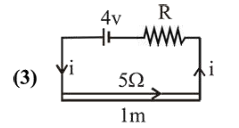# An ideal battery of 4 V and resistance RQuestion:

An ideal battery of $4 \mathrm{~V}$ and resistance $\mathrm{R}$ are connected in series in the primary circuit of a potentionmeter of length $1 \mathrm{~m}$ and resistance $5 \Omega$. The value of $\mathrm{R}$, to give a potential difference of $5 \mathrm{mV}$ across $10 \mathrm{~cm}$ of potentiometer wire is:

1. (1) $490 \Omega$

2. (2) $480 \Omega$

3. (3) $395 \Omega$

4. (4) $495 \Omega$

Correct Option: , 3

Solution:Current flowing through the circuit (I) is given by

$I=\left(\frac{4}{R+5}\right) A$

Resistance of length $10 \mathrm{~cm}$ of wire

$=5 \times \frac{10}{100}=0.5 \Omega$

According to question,

$5 \times 10^{-3}=\left(\frac{4}{R+5}\right) \cdot(0.5)$

$\therefore \frac{4}{\mathrm{R}+5}=10^{-2}$ or $\mathrm{R}+5=400 \Omega$

$\therefore \mathrm{R}=395 \Omega$